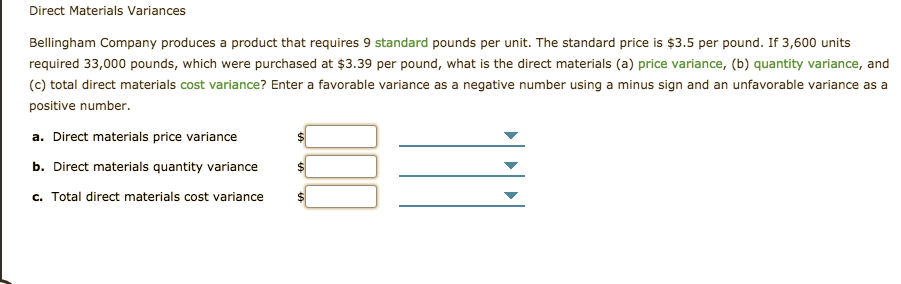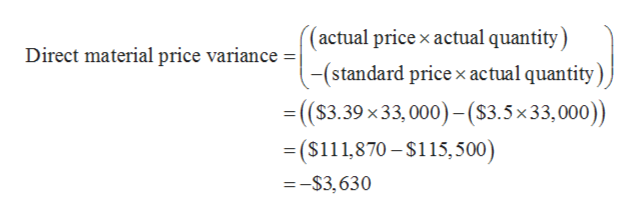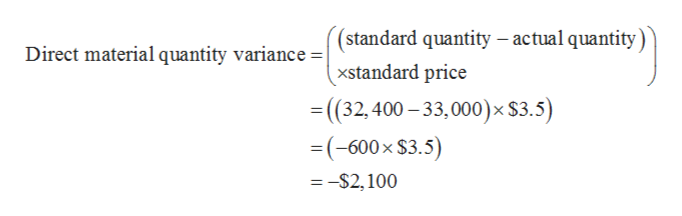# Direct Materials VariancesBellingham Company produces a product that requires 9 standard pounds per unit. The standard price is \$3.5 per pound. If 3,600 unitsrequired 33,000 pounds, which were purchased at \$3.39 per pound, what is the direct materials (a) price variance, (b) quantity variance, and(c) total direct materials cost variance? Enter a favorable variance as a negative number using a minus sign and an unfavorable variance as apositive numbera. Direct materials price varianceb. Direct materials quantity variancec. Total direct materials cost variance

Question
46 viewshelp_outlineImage TranscriptioncloseDirect Materials Variances Bellingham Company produces a product that requires 9 standard pounds per unit. The standard price is \$3.5 per pound. If 3,600 units required 33,000 pounds, which were purchased at \$3.39 per pound, what is the direct materials (a) price variance, (b) quantity variance, and (c) total direct materials cost variance? Enter a favorable variance as a negative number using a minus sign and an unfavorable variance as a positive number a. Direct materials price variance b. Direct materials quantity variance c. Total direct materials cost variance fullscreen
check_circle

Step 1

Variance is referred as the difference. In calculating price variance, a difference in the standard price of material and its actual price is calculated. In calculating quantity variance, difference between the standard quantity and actual quantity usage is calculated. Variance can be favorable or unfavorable.

Step 2

Actual quantity: 33,000 pounds

Actual price: \$3.39 per pound

Standard price: \$3.5 per pound

Standard quantity: (3,600×9) = 32,400

a)

Direct material price variance is calculated as follows:help_outlineImage Transcriptionclose(actual price x actual quantity) -(standard price x actual quantity) Direct material price variance = | -(\$3.39x33,000)-(\$3.5x33,000)) = (\$111,870-\$115,500) --\$3,630 fullscreen
Step 3

b)

Direct material quantity variance is cal...help_outlineImage Transcriptionclose(standard quantity - actual quantity) xstandard price (32,400-33,000)x\$3.5) = (-600x \$3.5) Direct material quantity variance =| = -\$2,100 fullscreen

### Want to see the full answer?

See Solution

#### Want to see this answer and more?

Solutions are written by subject experts who are available 24/7. Questions are typically answered within 1 hour.*

See Solution
*Response times may vary by subject and question.
Tagged in